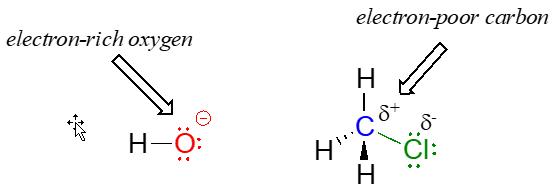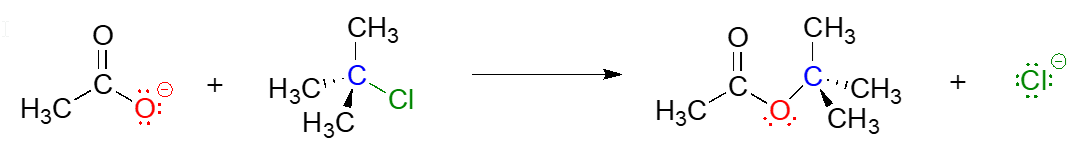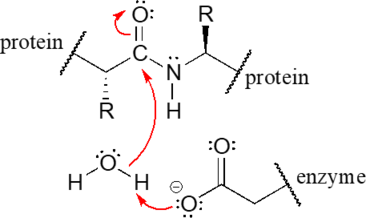# 5.2: A First Look at Some Organic Reaction Mechanisms

$$\newcommand{\vecs}{\overset { \rightharpoonup} {\mathbf{#1}} }$$ $$\newcommand{\vecd}{\overset{-\!-\!\rightharpoonup}{\vphantom{a}\smash {#1}}}$$$$\newcommand{\id}{\mathrm{id}}$$ $$\newcommand{\Span}{\mathrm{span}}$$ $$\newcommand{\kernel}{\mathrm{null}\,}$$ $$\newcommand{\range}{\mathrm{range}\,}$$ $$\newcommand{\RealPart}{\mathrm{Re}}$$ $$\newcommand{\ImaginaryPart}{\mathrm{Im}}$$ $$\newcommand{\Argument}{\mathrm{Arg}}$$ $$\newcommand{\norm}{\| #1 \|}$$ $$\newcommand{\inner}{\langle #1, #2 \rangle}$$ $$\newcommand{\Span}{\mathrm{span}}$$ $$\newcommand{\id}{\mathrm{id}}$$ $$\newcommand{\Span}{\mathrm{span}}$$ $$\newcommand{\kernel}{\mathrm{null}\,}$$ $$\newcommand{\range}{\mathrm{range}\,}$$ $$\newcommand{\RealPart}{\mathrm{Re}}$$ $$\newcommand{\ImaginaryPart}{\mathrm{Im}}$$ $$\newcommand{\Argument}{\mathrm{Arg}}$$ $$\newcommand{\norm}{\| #1 \|}$$ $$\newcommand{\inner}{\langle #1, #2 \rangle}$$ $$\newcommand{\Span}{\mathrm{span}}$$$$\newcommand{\AA}{\unicode[.8,0]{x212B}}$$

## An acid-base (proton transfer) reaction

We'll begin with a relatively simple type of reaction that you are no doubt familiar with from previous chemistry classes: an acid-base reaction. Note that in chapter 7, we will come back to consider acid-base reactivity in much greater detail. Here is the acid-base reaction between hydroxide ion and hydrochloric acid:Note

A reaction such as the one above would of course include a spectator cation, such as sodium ($$\ce{Na^{+}}$$) or potassium ($$\ce{K^{+}}$$). In most of the reaction figures that we'll see throughout this book, the spectator ion is not shown in the interest of simplicity.

A proton is transferred from $$\ce{HCl}$$, the acid, to hydroxide ion, the base. The product is water (the conjugate acid of hydroxide ion) and chloride ion (the conjugate base of $$\ce{HCl}$$).

Despite its simplicity (and despite the fact that the reactants and products are inorganic rather than organic), this reaction allows us to consider for the first time many of the fundamental ideas of organic chemistry that we will be exploring in various contexts throughout this text.

One very important key to understanding just about any reaction mechanism is the concept of electron density, and how it is connected to the electron movement (bond-breaking and bond-forming) that occurs in a reaction. The hydroxide ion – specifically, the oxygen atom bearing the negative formal charge – has high electron density: it is electron-rich.The hydrogen atom in $$\ce{HCl}$$, on the other hand, has low electron density: it is electron-poor, because chlorine is more electronegative than hydrogen. As you might expect, an atom that is electron-rich is likely to be attracted to an atom that is electron-poor. As hydroxide and $$\ce{HCl}$$ move closer to each other, a new bond forms between oxygen and hydrogen, and the hydrogen-chlorine bond breaks. The end result is a water molecule and a chloride anion.

In organic chemistry terms, a reaction mechanism is a formalized description of how a reaction takes place - how we get, in other words, from reactants to products. Previously (section 2.3) , we saw how curved arrows were used to depict the ‘imaginary’ movement of two electrons when illustrating the conversion between two resonance contributors of the same molecule or ion (remember from that discussion that the conversion between two resonance contributors is not a reaction - it is merely an illustration of two different ways to draw the same molecule). The same curved arrow convention is used in mechanism drawings to show the electron movement that takes place in chemical reactions, where bonds are actually broken and formed. The mechanism for the $$\ce{HCl + OH}$$- reaction, for example, can be depicted by drawing two curved arrows.Arrow (a) in the mechanistic drawing originates at one of the lone pairs on the hydroxide oxygen and points to the ‘H’ symbol in hydrochloric acid, illustrating the ‘attack’ of the oxygen lone pair and subsequent formation of a new hydrogen-oxygen bond. Arrow (b) originates at the middle of hydrogen-chlorine bond and points to the ‘$$\ce{Cl}$$’ symbol, indicating that this bond is breaking: the two electrons that make the bond are ‘leaving’ and becoming a lone pair on chloride ion. Always keep in mind that these curved arrows by definition depict the movement of two electrons. (When we study radical reactions in chapter 17, we will see how to depict the movement of a single electron.)

When two (or more) curved arrows are drawn in the same figure of a mechanism, the intended meaning is that the electron movements being shown are occurring simultaneously. For example, in the figure above, the electron movement illustrated by arrow (a) ($$\ce{O-H}$$ bond formation) is occurring at the same time as the $$\ce{H-Cl}$$ bond breaking illustrated by arrow (b).

The transition state (TS) of a chemical step is a point at which bonds are in the process of breaking and/or forming. (More specifically, we will see below when discussing energy diagrams that the transition state is the point of highest energy in the chemical step). Transition states are illustrated by drawing the forming/breaking bonds as dotted lines, and are enclosed by brackets with the 'double-dagger' symbol. For example, the transition state in the acid-base reaction between hydroxide and $$\ce{HCl}$$ can be illustrated as:Notice in the drawing above that both the oxygen and the chlorine bear partial negative charges at the transition state: the formal charge on oxygen changes from -1 to 0 during the course of the reaction step, while the formal charge on chlorine changes from 0 to -1.

While it can sometimes be instructive to include a transition state drawing in an organic mechanism diagram, they are not 'obligatory' elements of such a diagram. When asked to draw a reaction mechanism in the exercises and problems in this book, you need not include TS drawings in your answer unless specifically directed to do so.

Exercise $$\PageIndex{1}$$

Draw electron movement arrows to illustrate mechanism of the acid-base reaction between acetic acid, $$\ce{CH3CO2H}$$, and ammonia, $$\ce{NH3}$$. Draw out the Lewis structures of reactants and products, including all lone pairs and formal charges. Include a transition state drawing in your mechanism.

Exercise $$\PageIndex{2}$$

Draw electron movement arrows to illustrate the mechanism of the reverse of the reaction in Exercise $$\PageIndex{1}$$: the acid-base reaction between acetate ion ($$\ce{CH_3CO_2^-}$$, acting as a base) and ammonium ($$\ce{NH_4^+}$$), acting as an acid). Again, draw out the Lewis structures of reactants and products, including all lone pairs and formal charges.

## A one-step nucleophilic substitution reaction

The reaction between hydroxide and $$\ce{HCl}$$ is a simple example of a Brønsted acid-base (proton transfer) reaction. We now continue our introduction to the essential ideas of organic reactivity with a different type of reaction in which bonds to a carbon atom are rearranged. Consider what might happen if a hydroxide ion encountered a chloromethane molecule. The hydroxide is an electron-rich species, and thus might be expected to act as a base and ‘attack’ a hydrogen as it did in the previous example with hydrochloric acid. In this case, though, the three hydrogens on chloromethane are not electron-poor, as they are bound not to chlorine but to carbon, which is not very electronegative. However, there is a relatively electron-poor atom in chloromethane: the carbon itself.Due to the relative electronegativity of chlorine, the carbon-chlorine bond is polar. It stands to reason that a lone pair of electrons on the electron-rich hydroxide oxygen will be attracted to the electron-poor carbon nucleus.In the mechanism drawing above, curved arrow (a) shows the lone pair electrons on the hydroxide oxygen moving to fill up an $$sp^3$$ orbital on chloromethane, forming a new carbon-oxygen s bond. However, in order for this new bond to form, one of the bonds already on the carbon must simultaneously break - otherwise, there will be five bonds to carbon and the octet rule will be violated (remember that the 'octet rule' tells us that elements in the second row of the periodic table can have a maximum of eight valence electrons). Curved arrow (b) illustrates how the two electrons in the carbon-chloride bond break out of their s bond and become a lone pair on the chloride ion product. In other words, arrow (b) illustrates the breaking of the carbon-chlorine bond. (We will see a transition state drawing for this reaction in chapter 8, when we study this type of reaction mechanism in much greater detail).

The reaction mechanism illustrated above called a nucleophilic substitution. The 'substitution' term is easy to understand: just recognize how hydroxide substitutes for chlorine as the fourth bond to the central carbon. The term 'nucleophilic' means 'nucleus-loving' and refers to the electron-rich species, the hydroxide oxygen. This oxygen is a nucleophile: it is electron-rich and attracted to the electron-poor nucleus of the carbon atom, and 'attacks' with a lone pair to form a new covalent bond.

There are two more terms that come into play here, both of which you will see again and again as you continue to study organic reactions. Because the carbon atom in methyl chloride is electron-poor, it is attracted to anything that is electron rich - anything nucleophilic, in other words. Thus, the carbon is referred to in this context as an electrophile. The chlorine, because it leaves with two electrons to become a chloride ion, is termed a leaving group.Exercise $$\PageIndex{3}$$

In each of the nucleophilic substitution reactions below, identify the nucleophile, electrophile, and leaving group, and fill in the missing product.## A Two-step Nucleophilic Substitution Mechanism

Reaction mechanisms describe not only the electron movement that occurs in a chemical reaction, but also the order in which bond-breaking and bond-forming events occur. Some nucleophilic substitution reactions, for example, can occur by a two-step mechanism that is different from the one-step mechanism we just saw between hydroxide ion and chloromethane. Look, for example, at the substitution reaction between acetate ion and 2-chloro-2-methyl propane (common name tert-butyl chloride).Unlike the chloromethane plus hydroxide reaction, in which the substitution process took place in a single, concerted step, it turns out that this mechanism involves two separate steps. The leaving group, chloride anion, leaves first, before the acetate nucleophile attacks.

Step 1: Loss of leaving group (slow)Step 2: Nucleophilic Attack (fast)Because the central carbon (colored blue in the figure above) has lost its share of the two electrons in what was the carbon-chlorine bond, it is now positively charged. Recall from section 2.1 that we can picture a carbocation as a planar, $$sp^2$$-hybridized carbon center with three bonds, an empty $$p$$ orbital, and a full positive charge.The carbocation is highly reactive, and does not exist for very long before participating in a subsequent bond-forming event. In the language of organic mechanisms, it is referred to as a reaction intermediate.

With its empty $$p$$ orbital, the carbocation intermediate is clearly electron-poor, and thus is a powerful electrophile. The negatively charged acetate ion is electron dense and a nucleophile, and as such is strongly attracted to the carbocation electrophile. Attack by the nucleophile results in a new carbon-oxygen s bond and formation of the substitution product.

We will have much more to say about nucleophilic substitutions, nucleophiles, electrophiles, and leaving groups in chapter 8. The take home message at this point, however, is simply that two reactions that look quite similar in terms of the reactants and products can occur by different mechanisms.

Curved Arrows

You can probably appreciate by now how essential it is to understand and be able to work with curved arrows - it is something that will use constantly during the remainder of your study of organic reactivity.

For example, although you are not yet familiar with the relevant reaction mechanism (it is the HIV protease reaction, covered in chapter 11), given reactant and intermediate structures:with practice you should at this point be able to recognize the bond-forming and bond-breaking electron movement that is taking place, and draw the appropriate curved arrows:An additional word of caution: many beginning organic students make the mistake of using curved arrows to depict the motion of atoms. This is incorrect! The curved arrows in an organic mechanism always refer to the motion of electrons.

## Contributors

This page titled 5.2: A First Look at Some Organic Reaction Mechanisms is shared under a CC BY-NC-SA 4.0 license and was authored, remixed, and/or curated by Tim Soderberg via source content that was edited to the style and standards of the LibreTexts platform; a detailed edit history is available upon request.